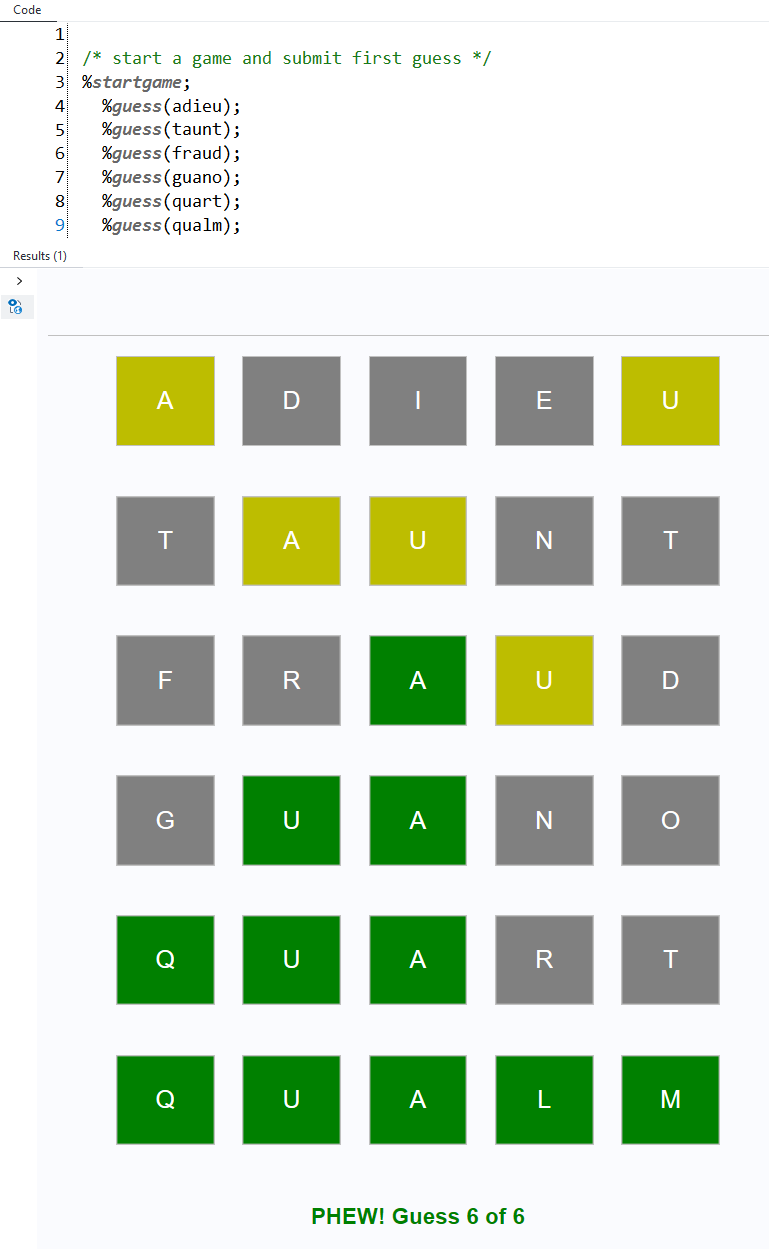Instantly share code, notes, and snippets.

# huytd/wordle.md

Last active October 9, 2023 02:23
Star You must be signed in to star a gist
Wordle in less than 50 lines of Bash
This file contains bidirectional Unicode text that may be interpreted or compiled differently than what appears below. To review, open the file in an editor that reveals hidden Unicode characters. Learn more about bidirectional Unicode characters
 words=(\$(grep '^\w\w\w\w\w\$' /usr/share/dict/words | tr '[a-z]' '[A-Z]')) actual=\${words[\$[\$RANDOM % \${#words[@]}]]} end=false guess_count=0 max_guess=6 if [[ \$1 == "unlimit" ]]; then max_guess=999999 fi while [[ \$end != true ]]; do guess_count=\$(( \$guess_count + 1 )) if [[ \$guess_count -le \$max_guess ]]; then echo "Enter your guess (\$guess_count / \$max_guess):" read guess guess=\$(echo \$guess | tr '[a-z]' '[A-Z]') if [[ " \${words[*]} " =~ " \$guess " ]]; then output="" remaining="" if [[ \$actual == \$guess ]]; then echo "You guessed right!" for ((i = 0; i < \${#actual}; i++)); do output+="\033[30;102m \${guess:\$i:1} \033[0m" done printf "\$output\n" end=true else for ((i = 0; i < \${#actual}; i++)); do if [[ "\${actual:\$i:1}" != "\${guess:\$i:1}" ]]; then remaining+=\${actual:\$i:1} fi done for ((i = 0; i < \${#actual}; i++)); do if [[ "\${actual:\$i:1}" != "\${guess:\$i:1}" ]]; then if [[ "\$remaining" == *"\${guess:\$i:1}"* ]]; then output+="\033[30;103m \${guess:\$i:1} \033[0m" remaining=\${remaining/"\${guess:\$i:1}"/} else output+="\033[30;107m \${guess:\$i:1} \033[0m" fi else output+="\033[30;102m \${guess:\$i:1} \033[0m" fi done printf "\$output\n" fi else echo "Please enter a valid word with 5 letters!"; guess_count=\$(( \$guess_count - 1 )) fi else echo "You lose! The word is:" echo \$actual end=true fi done

### BMDan commented Feb 9, 2022 • edited

As promised, the version without arrays. Also makes some minor stylistic changes, deletes an unnecessary loop, and adds an "abandon" feature (press CTRL-D at the prompt). 36 lines, total.

```actual="\$(sort -R /usr/share/dict/words | grep -xEm 1 '\w{5}' | tr '[:lower:]' '[:upper:]')"
guess_count=0 max_guess=6
[[ "\${1//unlimit}" != "\${1:-}" ]] && max_guess=999999
while true; do
guess_count=\$(( guess_count + 1 ))
if [[ \$guess_count -le \$max_guess ]]; then
while read -r -p "Enter your guess (\$guess_count / \$max_guess): " guess; do
grep -ixF "\${guess:-inv.alid}" /usr/share/dict/words | grep -xqE '\w{5}' && break
[[ \${#guess} != 5 ]] && echo "Too short/long." && continue
echo "Not a real word."
done
[ \${#guess} -eq 0 ] && echo && echo "Giving up so soon?  The answer was \$actual." && break
guess="\$(tr '[:lower:]' '[:upper:]' <<<"\$guess")"
output="" remaining=""
for ((i = 0; i < \${#actual}; i++)); do
[[ "\${actual:\$i:1}" != "\${guess:\$i:1}" ]] && remaining+=\${actual:\$i:1}
done
for ((i = 0; i < \${#actual}; i++)); do
if [[ "\${actual:\$i:1}" != "\${guess:\$i:1}" ]]; then
if [[ "\$remaining" == *"\${guess:\$i:1}"* ]]; then
output+="\$(tput setaf 0)\$(tput setab 11) \${guess:\$i:1} \$(tput sgr0)"
remaining=\${remaining/"\${guess:\$i:1}"/}
else
output+="\$(tput setaf 0)\$(tput setab 15) \${guess:\$i:1} \$(tput sgr0)"
fi
else
output+="\$(tput setaf 0)\$(tput setab 10) \${guess:\$i:1} \$(tput sgr0)"
fi
done
echo "\$output"
[ "\$actual" = "\$guess" ] && echo "You guessed right!" && break
else
echo "You lose!  The word was \$(tput setaf 1)\$(tput bold)\$actual\$(tput sgr0)."
break
fi
done```

### kristinbell commented Mar 12, 2022

Here's a challenge for someone: Can we make a game that uses more than one language at a time? Would that just involve using two dictionaries? Or would the game need different rules?

### cjdinger commented Mar 17, 2022

I wrote a SAS version and placed it here sascommunities/wordle-sas. Uses the word lists from cfreshman (thanks) and arrays to check guesses.